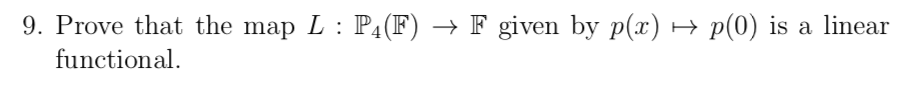# 9. Prove that the map L: P4(F) → F given by p(x) →functional.p(0) is a linear

Question
1 viewshelp_outlineImage Transcriptionclose9. Prove that the map L: P4(F) → F given by p(x) → functional. p(0) is a linear fullscreen
check_circle

Step 1

We are given that L(p(x)) = p(0).

Let p(x), q(x) in P4(F) and α, β in the field F.

Now,

L( αp(x) + βq(x) ) = L( (αp+βq)(x) )

= (αp+βq)(0)

&n...

### Want to see the full answer?

See Solution

#### Want to see this answer and more?

Solutions are written by subject experts who are available 24/7. Questions are typically answered within 1 hour.*

See Solution
*Response times may vary by subject and question.
Tagged in